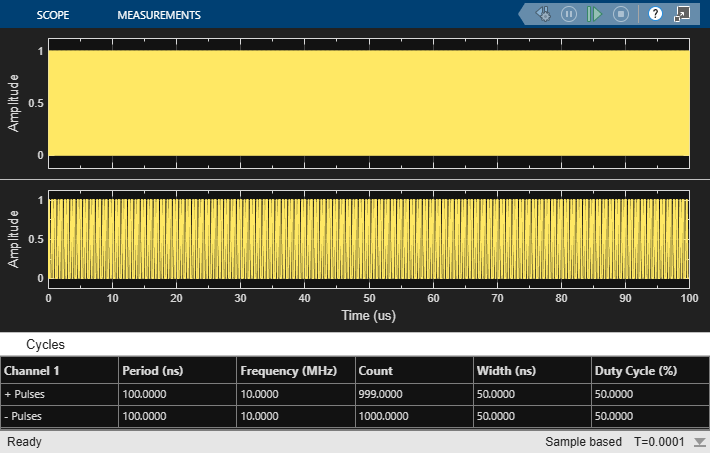# Frequency Division Using Dual Modulus Prescaler

Open the model `Dual_Modulus_Prescaler_Ex`. The model consists of a Pulse Generator and a Dual Modulus Prescaler block.

```model='Dual_Modulus_Prescaler_Ex'; open_system(model) ```The period of the incoming pulse at the clk in port is `1e-7` s. So, the incoming signal has a frequency of `10` MHz. The Program counter value, Prescaler divider value, and Swallow counter value are `4`, `1`, and `1`, respectively. The effective clock divider value of the dual modulus prescaler is `5`.

Run the simulation for `1e-4` s. The frequency of the output signal is `2.002` MHz.

```sim(model); ```